Electron. J. Diff. Equ., Vol. 2011 (2011), No. 145, pp. 1-11.

### Asymptotically linear fourth-order elliptic problems whose nonlinearity crosses several eigenvalues Evandro Monteiro

Abstract:
In this article we prove the existence of multiple solutions for the fourth-order elliptic problem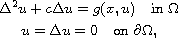where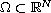is a bounded domain,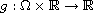is a function of class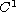such that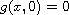and it is asymptotically linear at infinity. We study the cases when the parameter c is less than the first eigenvalue, and between two consecutive eigenvalues of the Laplacian. To obtain solutions we use the Saddle Point Theorem, the Linking Theorem, and Critical Groups Theory.

Submitted February 15, 2011. Published November 2, 2011.
Math Subject Classifications: 35J30, 35J35.
Key Words: Asymptotically linear; Morse theory; shifting theorem; multiplicity of solutions.

Show me the PDF file (231 KB), TEX file, and other files for this article.Evandro Monteiro UNIFAL-MG, Rua Gabriel Monteiro da Silva, 700. Centro CEP 37130-000 Alfenas-MG, Brazil email: evandromonteiro@unifal-mg.edu.br